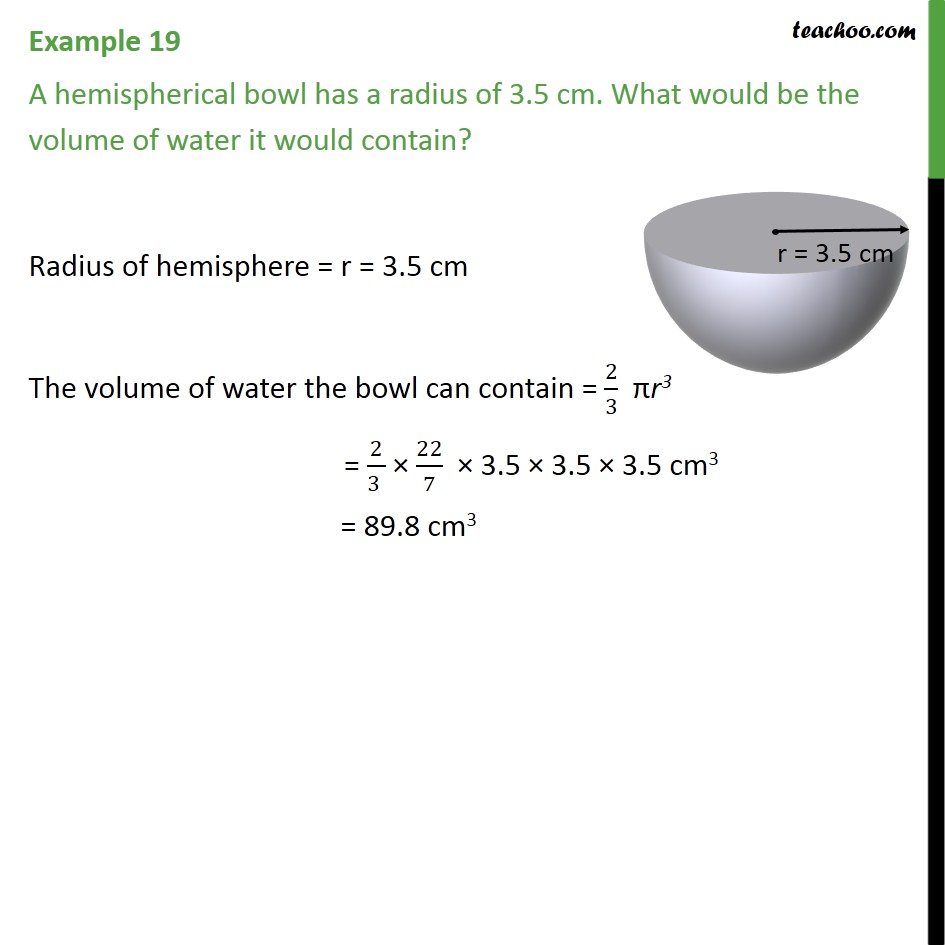1. Chapter 13 Class 9 Surface Areas and Volumes (Term 2)
2. Concept wise
3. Volume Of Hemisphere

Transcript

Example 19 A hemispherical bowl has a radius of 3.5 cm. What would be the volume of water it would contain? Radius of hemisphere = r = 3.5 cm The volume of water the bowl can contain = 2/3 πr3 = 2/(3 ) × 22/7 × 3.5 × 3.5 × 3.5 cm3 = 89.8 cm3